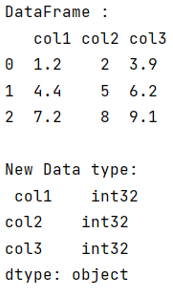# Python - Convert entire pandas dataframe to integers

Given a Pandas DataFrame, we have to convert it into integers.
Submitted by Pranit Sharma, on August 04, 2022

Pandas is a special tool that allows us to perform complex manipulations of data effectively and efficiently. Inside pandas, we mostly deal with a dataset in the form of DataFrame. DataFrames are 2-dimensional data structures in pandas. DataFrames consist of rows, columns, and data.

There are multiple data types that are supported by pandas,

• Int
• Float
• Object
• Boolean
• Datetime

All these data types can be converted into some other data types using the astype() method.

This method is used when we want to convert the data type of one single column or a series, but if we want to convert the entire DataFrame, for this purpose, we have a method called pandas.to_numeric() method but again it fails in case of float values and hence we have to loop over the columns of the DataFrame to change the data type to float first then we will convert them to int.

Let us understand with the help of an example,

## Python code to convert entire pandas dataframe to integers

```# Importing pandas package
import pandas as pd

# Creating a dictionary
d = {
'col1':['1.2','4.4','7.2'],
'col2':['2','5','8'],
'col3':['3.9','6.2','9.1']
}

# Creating a dataframe
df = pd.DataFrame(d)

# Display Dataframe
print("DataFrame :\n",df,"\n")

# Looping over df.columns
for i in df.columns:
try:
df[[i]] = df[[i]].astype(float).astype(int)
except:
pass

# Display data types of dataframe
print("New Data type:\n",df.dtypes)
```

Output: